# AP Statistics Multiple-Choice Practice Questions: Graphical Displays

### Test Information9 questions21 minutes

1. The stemplot below shows ages of CEOs of a select group of corporations.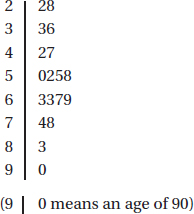2. Which of the following is a true statement?

3. Which of the following is an incorrect statement?

4. Question below refers to the following five cumulative relative frequency plots: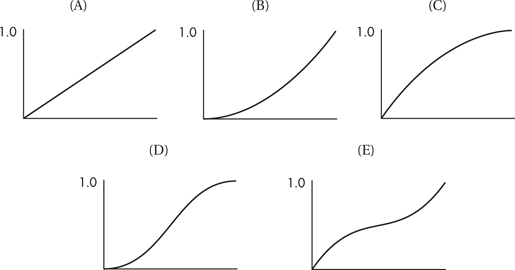To which of the above cumulative relative frequency plots does the following histogram correspond?5. Question below refers to the following five cumulative relative frequency plots:To which of the above cumulative relative frequency plots does the following histogram correspond?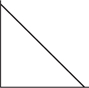6. Question below refers to the following five cumulative relative frequency plots:To which of the above cumulative relative frequency plots does the following histogram correspond?7. Question below refers to the following five cumulative relative frequency plots:To which of the above cumulative relative frequency plots does the following histogram correspond?8. Question below refers to the following five cumulative relative frequency plots:To which of the above cumulative relative frequency plots does the following histogram correspond?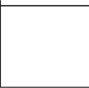9. An auto manufacturer claims that the autos it sells have an average fuel efficiency of 35 mpg. A consumer group believes that the true figure is lower. The consumer group obtains 5 of the company's autos and determines an average of 32 mpg. Is this significant? The company runs a simulation by randomly picking 5 cars 160 times from a fleet of cars of known 35 mpg efficiency and calculating the resulting averages to show that 32 was possible. The company makes the following dotplot.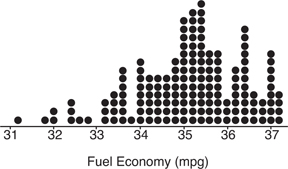Does the company's argument seem reasonable given this dotplot?